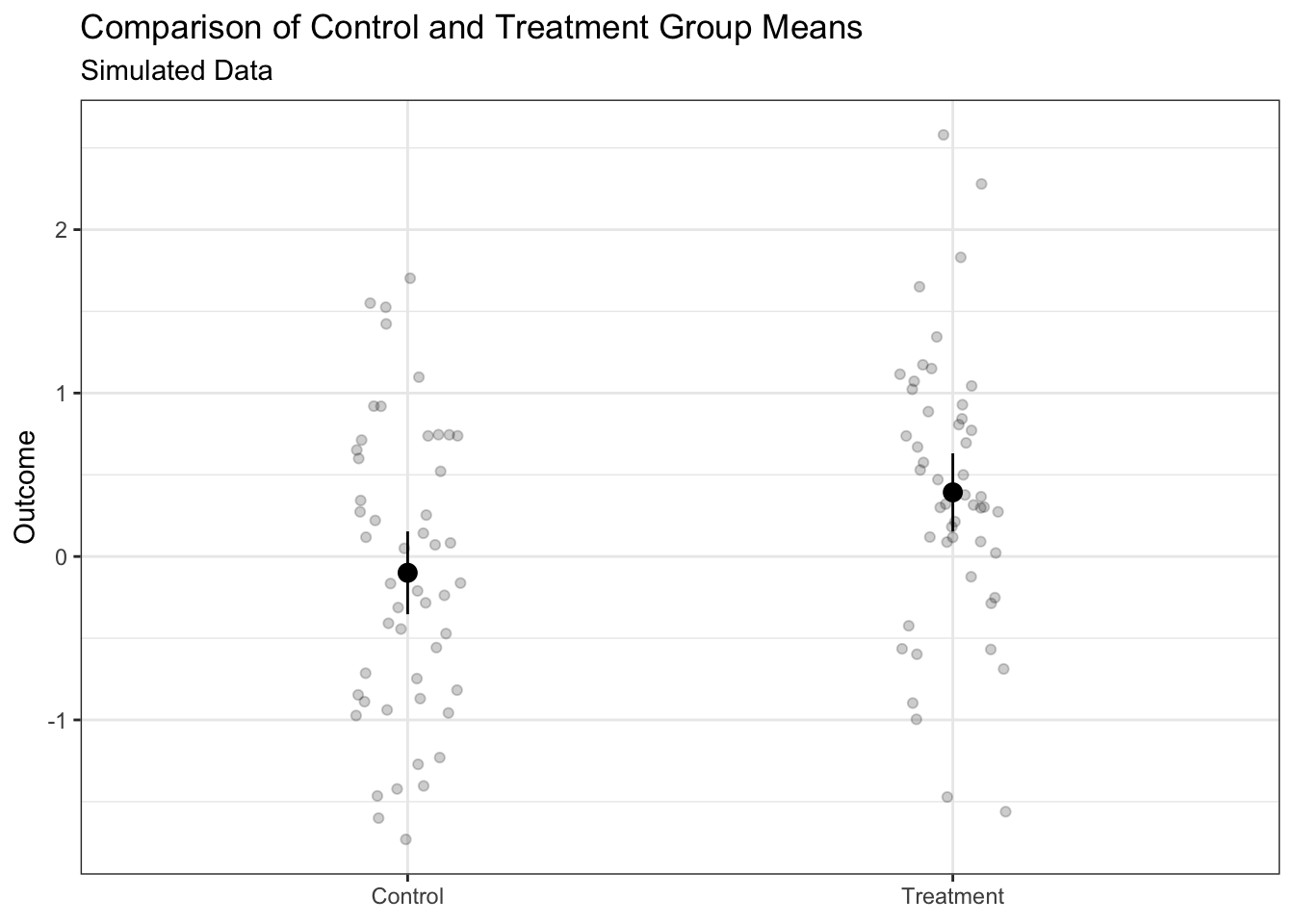This document shows some things researchers may wish to add to their preanalysis plans using design declaration and diagnosis.

# Setup

library(DeclareDesign)
library(tidyverse)
library(texreg)
library(knitr)
set.seed(343)

# Declare the Design

sample_size    <- 100
effect_size    <- 0.5
number_treated <- 50

two_arm_design <-

# M: Model
declare_population(N = sample_size, u = rnorm(N)) +
declare_potential_outcomes(Y ~ effect_size * Z + u) +

# I: Inquiry
declare_estimand(ATE = mean(Y_Z_1 - Y_Z_0)) +

# D: Data Strategy
declare_assignment(m = number_treated) +
declare_reveal(Y, Z) +

declare_estimator(Y ~ Z, model = lm_robust, estimand = "ATE")

# Mock Figure

one_run <- draw_data(two_arm_design)

one_run <-
one_run %>%
mutate(treatment = factor(Z, levels = 0:1, labels = c("Control", "Treatment")))

summary_df <-
one_run %>%
group_by(treatment) %>%
do(tidy(lm_robust(Y ~ 1, data = .))) %>%
mutate(Y = estimate)

ggplot(summary_df, aes(treatment, Y)) +
geom_point(size = 3) +
geom_errorbar(aes(ymin = conf.low, ymax = conf.high), width = 0) +
geom_point(data = one_run, position = position_jitter(width = 0.1), alpha = 0.2) +
theme_bw() +
theme(axis.title.x = element_blank()) +
ylab("Outcome") +
ggtitle("Comparison of Control and Treatment Group Means", "Simulated Data")# Mock Regression Table

fit <- lm_robust(Y ~ treatment, data = one_run)
htmlreg(
fit,
custom.coef.names = c("Intercept", "Treatment"),
include.ci = FALSE,
include.rmse = FALSE,
stars = 0.05,
custom.note = "%stars. HC2 robust standard errors are in parentheses.",
caption = "Average Treatment Effect (Simulated Data)",
caption.above = TRUE,
doctype = FALSE
)
Average Treatment Effect (Simulated Data)
Model 1
Intercept -0.10
(0.13)
Treatment 0.49*
(0.17)
R2 0.08
Num. obs. 100
*p < 0.05. HC2 robust standard errors are in parentheses.

# Diagnosis

diagnosis <- diagnose_design(two_arm_design)
reshape_diagnosis(diagnosis, select = "Power") %>%
kable()
Design Label Estimand Label Estimator Label Term N Sims Power
two_arm_design ATE estimator Z 500 0.73
(0.02)